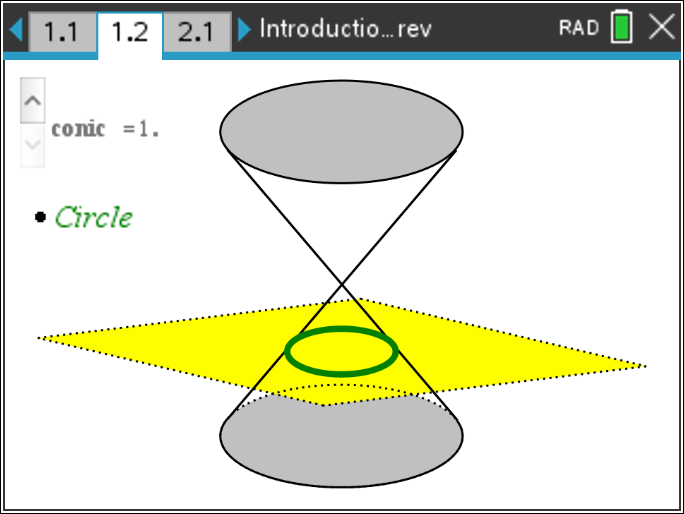••• ##### Device
•TI-Nspire™ CX/CX II
•TI-Nspire™ CX CAS/CX II CAS
• TI-Nspire™ Navigator™
• ##### Software

TI-Nspire™ CX
TI-Nspire™ CX CAS

5.0

• ##### Report an Issue

Precalculus: Introduction to Conic Sections
by Texas Instruments#### Objectives

• Students will identify how each conic results from slicing cones.
• Students will understand the locus definition of a parabola.
• Students will describe how the values of a, h, and k in the vertex form of the equation of a parabola affect its graph.
• Students will use the locus definition of a parabola to derive the equation of a parabola and will describe the relationships among the focus, the directrix, and the values in the vertex form of a parabola.

#### Vocabulary

• circle
• ellipse
• parabola
• hyperbola
• axis of symmetry
• focus
• directrix